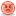CodeGuru Home VC++ / MFC / C++ .NET / C# Visual Basic VB Forums Developer.com

# Thread: assistance needed. Thanks

1.Junior MemberJoin Date
Oct 2010
Posts
2

##assistance needed. Thanks

Question to be solved:

1) 1. Create a program that will take an integer number as input from the user and store the number into a two dimensional array, show the user the location of the array that value will be going into. Once the array is full, loop through and display the elements to the screen and finally display the highest number that was entered into the array.

The array will look like this

const int MAX_A = 3;
const int MAX_B = 4;
//declare two dimensional array
int numbers[MAX_A][MAX_B];

my code:

it doesn't print the array values or the highest number... how can i get it to display?

how do i find the location of the elements inputted and display them?

#include<iostream>
#include<string>

const int MAX_A = 3;
const int MAX_B = 4;
//declare two dimensional array
int numbers[MAX_A][MAX_B];

int main()
{
// declaring variables
using namespace std;
int MAX_A = 3;
int MAX_B = 4;
int numberArray;
// asking the user to input data & storing in array
cout << "This program will take the user's input and store into an array." << endl;
cout << "Please enter any number, up to a series of 12 numbers..." << endl;
// declaring two-demensional array

for (int i=0; i < MAX_A; i++)
{
for (int j=0; j < MAX_B; j++)
{
while(1) // keep trying until we get a valid input
{
cout<<"Enter a number: "<<endl;
cin>>numberArray[i][j];
if (!cin)
{
cout << "Please enter a real, positive number." << endl;
// clean up the stream to remove the everything entered up to that point
}
else
break; // if we got a number, break the innermost loop
} // error checking loop
} // MAX_B loop
} // MAX_A loop
// print the array and the greatest value
for ( int i=0; i < MAX_A; i++) {
for (int j=0; j < MAX_B; j++){
cout << numberArray[i] [j] << "";
}
cout << endl;
}
system("PAUSE");
int highest = 0;
for ( int i=0; i< MAX_A; i++){
for (int j=0; j < MAX_B; j++){

// print the item
if (highest < numberArray[i][j])
highest = numberArray[i][j];
// print highest
cout << "Highest:" << highest << endl;
}
}

}Reply With Quote

2. ## Re: assistance needed. Thanks

Please put code tags around your code and properly indent it. Right now your code is very hard to read.Reply With Quote

3.Junior MemberJoin Date
Oct 2010
Posts
2

## Re: assistance needed. Thanks

it doesn't print the array values or the highest number... how can i get it to display?

how do i find the location of the elements inputted and display them?

<code>

#include<iostream>
#include<string>

const int MAX_A = 3;
const int MAX_B = 4;

//declare two dimensional array

int numbers[MAX_A][MAX_B];

int main()
{

// declaring variables

using namespace std;
int MAX_A = 3;
int MAX_B = 4;

// declaring two-demensional array

int numberArray;

// asking the user to input data & storing in array

cout << "This program will take the user's input and store into an array." << endl;
cout << "Please enter any number, up to a series of 12 numbers..." << endl;

for (int i=0; i < MAX_A; i++)
{
for (int j=0; j < MAX_B; j++)
{
while(1) // keep trying until we get a valid input
{
cout<<"Enter a number: "<<endl;
cin>>numberArray[i][j];
if (!cin)
{
cout << "Please enter a real, positive number." << endl;

// clean up the stream to remove the everything entered up to that point
}
else
break;
}
}
}

// print the array and the greatest value

for ( int i=0; i < MAX_A; i++)
{
for (int j=0; j < MAX_B; j++)
{
cout << numberArray[i] [j] << "";
}
cout << endl;
}
system("PAUSE");
int highest = 0;
for ( int i=0; i< MAX_A; i++){
for (int j=0; j < MAX_B; j++){

// print the item
if (highest < numberArray[i][j])
highest = numberArray[i][j];
// print highest
cout << "Highest:" << highest << endl;
}
}

}

</code>Reply With Quote

#### Tags for this Thread

array, c++, int array, integers, number####Posting Permissions

• You may not post new threads
• You may not post replies
• You may not post attachments
• You may not edit your posts
•

Click Here to Expand Forum to Full Width

Featured